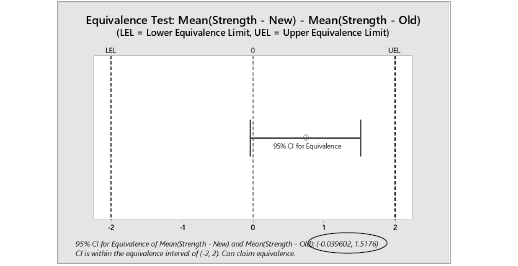STAT-16: Statistical Techniques for Equivalence Testing

This is part of a series of articles covering the procedures in the book Statistical Procedures for the Medical Device Industry.

Purpose

This procedure provides statistical techniques for demonstrating equivalence of a new product or process to an existing product or process. This is an alternate approach to demonstrating the product or process meets established requirements per the following procedures:.

Appendices

1. Confidence Limits for the Difference between Two Averages
2. Equivalence Test for Two Averages
3. Confidence Limits for the Difference between Two Averages—Paired Data
4. Equivalence Test for Two Averages—Paired Data
5. Confidence Limits for the Ratio of Two Standard Deviations
6. Equivalence Test for Two Standard Deviations

Highlights

• Equivalence does not mean identical.  It means the difference is less than some predetermined difference Δ.

• Demonstrating equivalence requires defining a difference Δ that is considered significant and then demonstrating with high confidence the difference is less than Δ.  Equivalence tests are based on confidence intervals.  The validated spreadsheet STAT-12 to 16 – Confidence Intervals and Equivalence Tests.xlsx  accompanying the book can be used for performing equivalence tests.  For example, suppose a difference of 2 or more is considered a significant difference.  As shown below, the 95 confidence interval for the difference is between -2 and 2 so one can claim equivalence.• Procedures for calculating sample size are provided.  However, a passing equivalence test is valid regardless of the sample size used.  For smaller sample sizes the confidence intervals will be wider, making it harder to pass.  The risk of too small a sample size is falsely failing the equivalence test.  The procedure for calculating sample size is too ensure a reasonable chance of passing equivalent groups.
• Equivalence tests cannot be chained together.  B is equivalent to A and C is equivalent to B does not mean C is equivalent to A.  The difference could be 2 times Δ.  One must prove directly that C is equivalent to A.  An alternate approach is to use A to establish specification limits and then show B and C meet the specification limits.  Setting specification limits is described in STAT-11, Statistical Techniques for Setting Specifications.
• A t-test by itself is not a valid approach for demonstrating equivalence.  If it is believed that equivalence testing requires fewer samples than demonstrating the specification limits are meet, equivalence testing is being done wrong.  Equivalence testing is generally used when there are not specification limits.

4 thoughts on “STAT-16: Statistical Techniques for Equivalence Testing”

1.Thanks for all clear text! I wonder if we can also demonstrate equivalence with individual measurements instead of averages

1.I interpret demonstrating individual values are equivalent means to demonstrate they have equivalent distributions. One approach is to demonstrate both their averages and standard deviations are equivalent, assuming they are both normal distributions. Equivalency can also be defined in terms of Ppk< \sub>.

STAT-16 begins by trying and talk you out of performing side-by-side equivalency testing. Instead, historical data can be used to set specifications limits for individuals values as described in STAT-11, Statistical Techniques for Setting Specifications. Sampling plans for proportions from STAT-12 can then be used to demonstrate individuals values meet the specification limits. This is the approach to demonstrating equivalency to historical data I commonly recommend.

2.Thank you for presenting this topic!
As you have mentioned above, demonstrating equivalence requires defining a difference Δ. In pharma, where the technique (bioequivalency) is well established, this difference Δ is defined as acceptable difference between the products without clinical impact which is mostly supported e.g. by clinical research results.
What are the requirements in your opinion for justifying such difference in medical device verification tests without a specific specification available?
Do you always prefer historical data to set specifications first? What if the information about the predicate is limited (produced by another company)?
Is it possible to choose the difference on a pure statistical rationale?
What in addition would be required to avoid predicate creep?

1.In general, Δ is a difference between the products without clinical/functional impact. It is defined in terms of impact on the customer using clinical or user experience. There is not a purely statistical rationale. Basing it on specification limits assumes the specification limits define a region/difference where differences have little clinical/functional impact. If historical data is used to set a specification limit, it assumes the past performance is acceptable.

To avoid predicate creep, use historical or test data on the predicate device to establish limits. Otherwise, side-by-side comparisons always should be made back to the original predicate device, which at some point, becomes impossible.

Scroll to Top